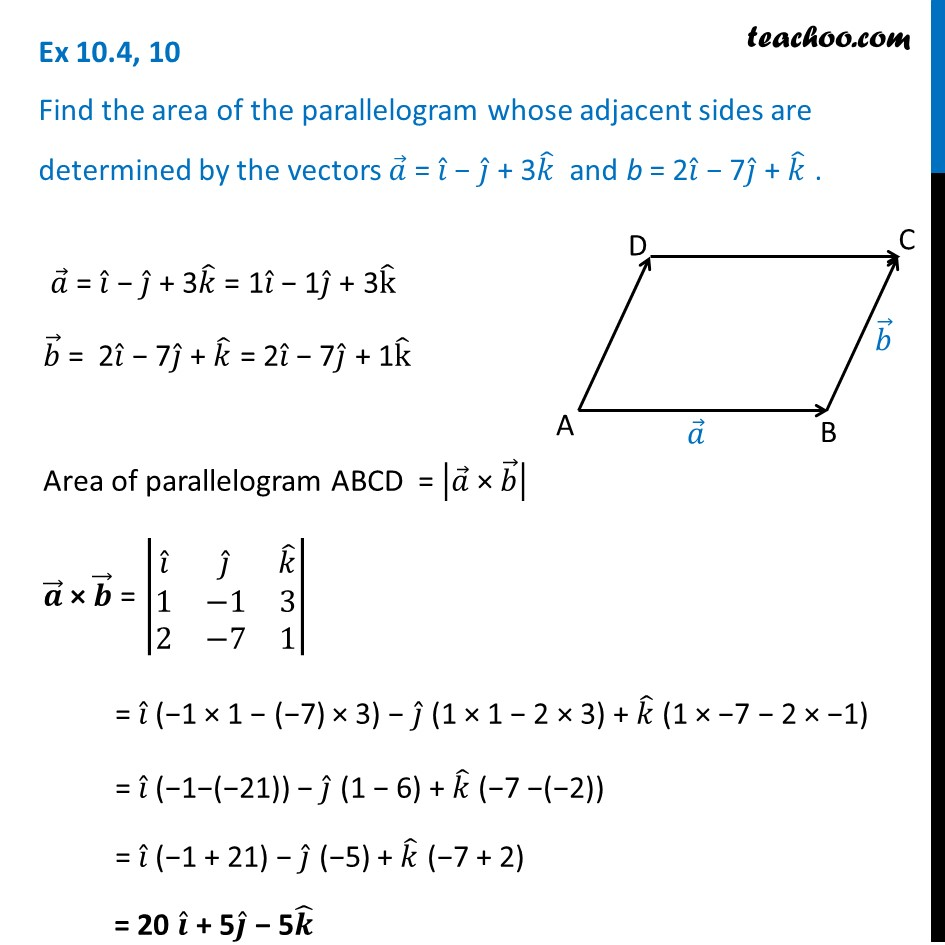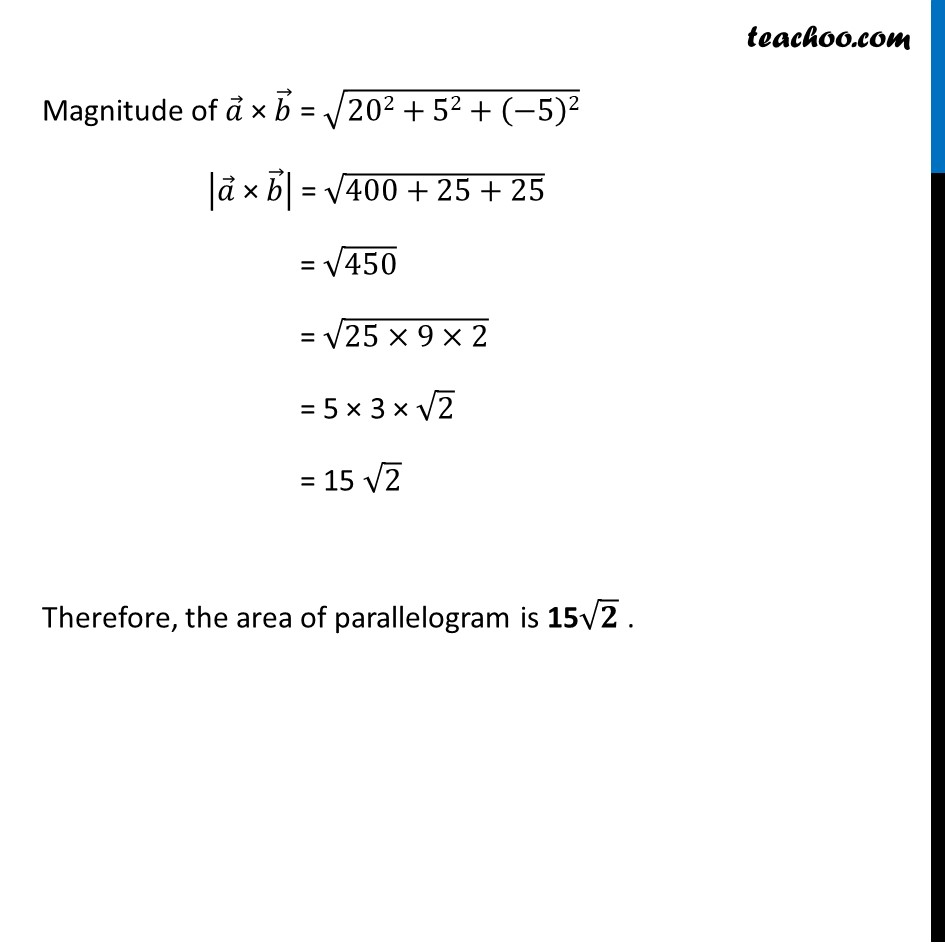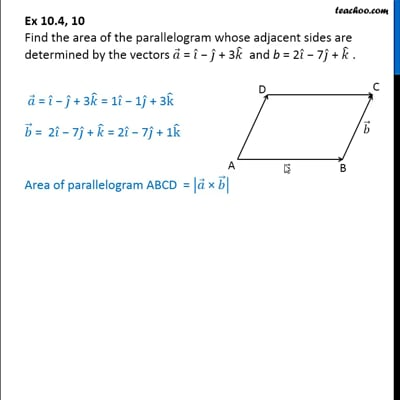Vector product - Area

Chapter 10 Class 12 Vector Algebra
Concept wiseThis video is only available for Teachoo black users

Introducing your new favourite teacher - Teachoo Black, at only ₹83 per month

### Transcript

Ex 10.4, 10 Find the area of the parallelogram whose adjacent sides are determined by the vectors 𝑎 ⃗ = 𝑖 ̂ − 𝑗 ̂ + 3𝑘 ̂ and b = 2𝑖 ̂ − 7𝑗 ̂ + 𝑘 ̂ . 𝑎 ⃗ = 𝑖 ̂ − 𝑗 ̂ + 3𝑘 ̂ = 1𝑖 ̂ − 1𝑗 ̂ + 3k ̂ 𝑏 ⃗ = 2𝑖 ̂ − 7𝑗 ̂ + 𝑘 ̂ = 2𝑖 ̂ − 7𝑗 ̂ + 1k ̂ Area of parallelogram ABCD = |𝑎 ⃗" × " 𝑏 ⃗ | 𝒂 ⃗ × 𝒃 ⃗ = |■8(𝑖 ̂&𝑗 ̂&𝑘 ̂@1&−1&3@2&−7&1)| = 𝑖 ̂ (−1 × 1 − (−7) × 3) − 𝑗 ̂ (1 × 1 − 2 × 3) + 𝑘 ̂ (1 × −7 − 2 × −1) = 𝑖 ̂ (−1−(−21)) − 𝑗 ̂ (1 − 6) + 𝑘 ̂ (−7 −(−2)) = 𝑖 ̂ (−1 + 21) − 𝑗 ̂ (−5) + 𝑘 ̂ (−7 + 2) = 20 𝒊 ̂ + 5𝒋 ̂ − 5𝒌 ̂ Magnitude of 𝑎 ⃗ × 𝑏 ⃗ = √(202+52+(−5)2) |𝑎 ⃗" × " 𝑏 ⃗ | = √(400+25+25) = √450 = √(25×9×2) = 5 × 3 × √2 = 15 √2 Therefore, the area of parallelogram is 15√𝟐 .